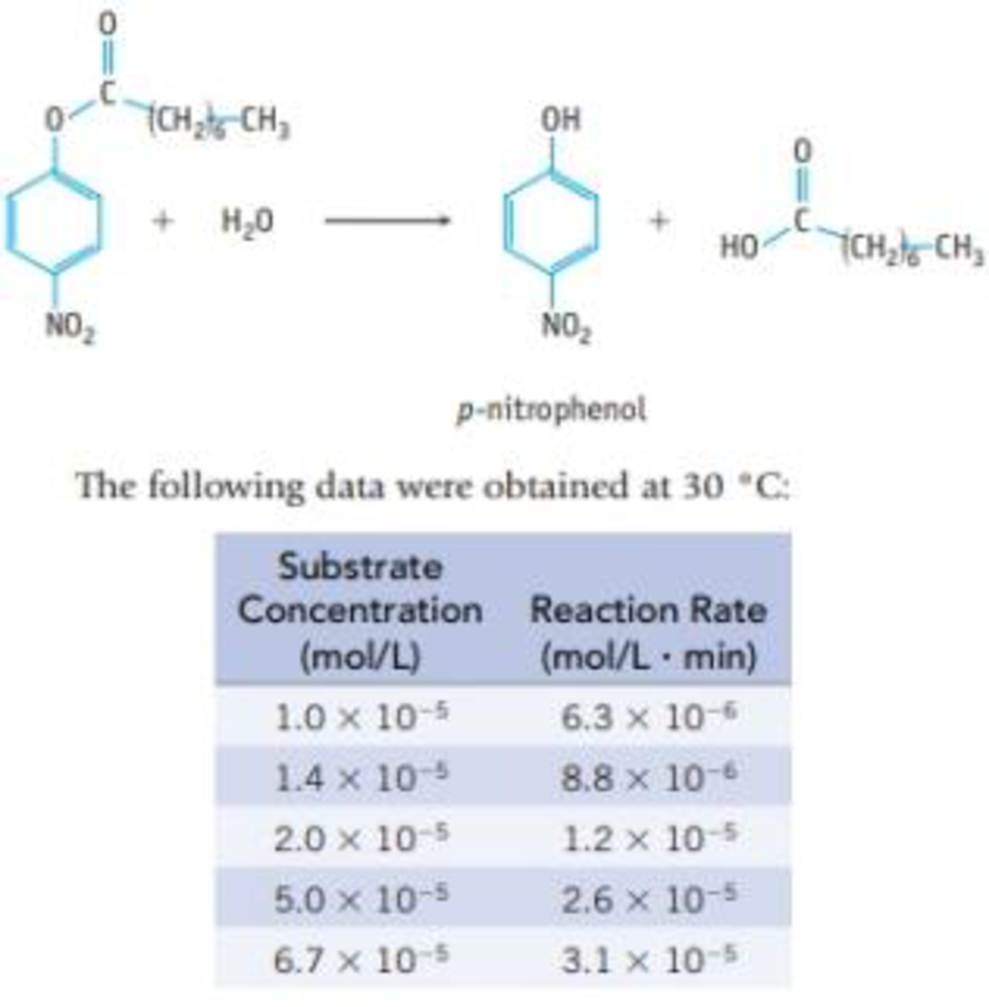Chapter 24, Problem 35IL

Chapter
Section
Textbook Problem

The enzyme lipase catalyzes the hydrolysis of esters of fatty acids. The hydrolysis of p-nitrophenyloctanoate was followed by measuring the appearance of p-nitrophenol in the reaction mixture:This reaction obeys Michaelis-Menten kinetics (Section 14.6). Determine the value of Ratemax for this reaction using the method described in Study Question 14.45.

Interpretation Introduction

Interpretation:

The value of Ratemax for the given reaction has to be determined.

Concept introduction:

For enzymatic reaction, the common equation is given below,

E+SESE+P

Here,

E is the enzyme.

S is the substrate.

ES is the enzyme substrate complex.

P is the product.

The general equation for Michaelis-Menten is dervied from above equation,

v°=Vmax([S][S]+Km)                                                                                      (1)

Here,

v° is the initial rate of the reaction.

Vmax is the rate maximum of the reaction.

S is the substrate concentration.

Km is the Michaelis-Menten constant which is half of the maximum velocity of the reaction.

Take the Reciprocal of both the sides in equation (1),

1v°=(KmVmax)(1[S])+1Vmax (2)

This equation is called as Lineweaver-Burk equation. By using this equation the value of Ratemax is calculated for the given reaction.

Explanation

The Ratemax for the given reaction is calculated below,

Given:

The substare concentration and reaction rate are given.

By using the equation,

1v°=(KmVmax)(1[S])+1Vmax

Calculate the value of 1v° and 1[S],

The graph between 1v° and 1[S] is plotted by taking the values from table (1).

The equation for the straight line obtained from the graph is y=0.148x+0.0092.

1v°×106=0.148×105x+1Vmax

Rearrange the equation, in form of y = mx + c.

y=1

Still sussing out bartleby?

Check out a sample textbook solution.

See a sample solution

The Solution to Your Study Problems

Bartleby provides explanations to thousands of textbook problems written by our experts, many with advanced degrees!

Get Started

Find more solutions based on key concepts